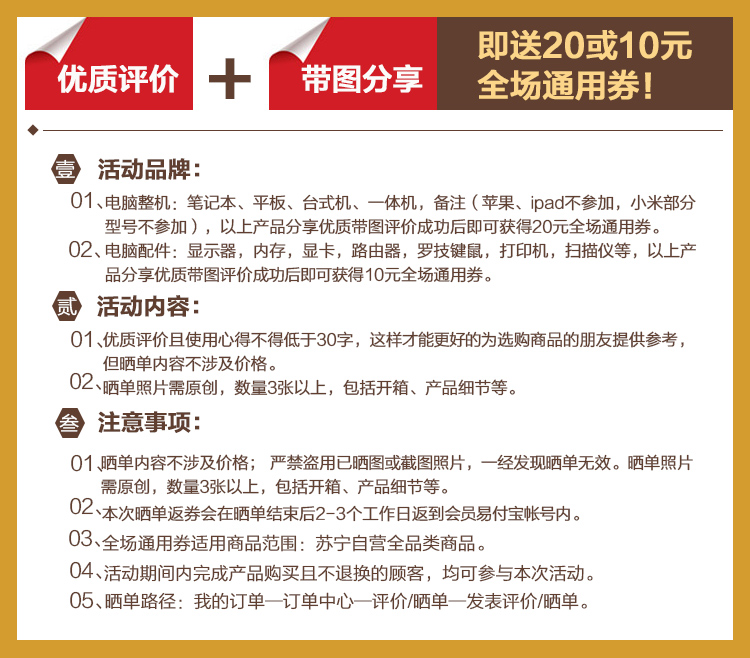•### 惠普（HP）ENVY 13-ad109TU笔记本电脑 var serverTime =1593680047 * 1000; \$(function(){ var dateTime = new Date(); var difference = dateTime.getTime() - serverTime; setInterval(function(){ \$(".endtime").each(function(){ var obj = \$(this); var endTime = new Date(parseInt(obj.attr('value')) * 1000); var nowTime = new Date(); var nMS=endTime.getTime() - nowTime.getTime() + difference; var myD=Math.floor(nMS/(1000 * 60 * 60 * 24)); var myH=Math.floor(nMS/(1000*60*60)) % 24; var myM=Math.floor(nMS/(1000*60)) % 60; var myS=Math.floor(nMS/1000) % 60; var myMS=Math.floor(nMS/100) % 10; if(myD>= 0){ var str ="<span>"+ myD+"</span>天<span>"+myH+"</span>小时<span>"+myM+"</span>分<span>"+myS+"."+myMS+"</span>秒"; }else{ var str = "已结束！"; } obj.html(str); }); }, 100); });CopyRight @ yershop 2014 - 2020 鄂ICP备15013601号-1 ';